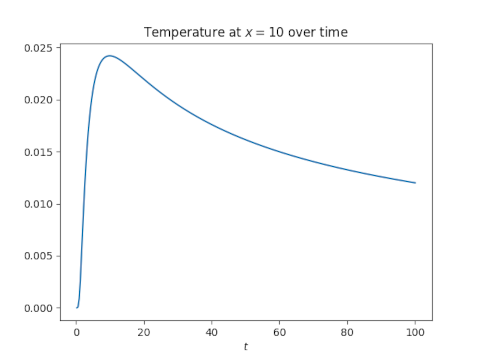# Heat equation and the normal distribution

The density function of a normal distribution with mean 0 and standard deviation √(2kt) satisfies the heat equation. That is, the functionsatisfies the partial differential equationYou could verify this by hand, or if you’d like, here’s Mathematica code to do it.

      u[x_, t_] := PDF[NormalDistribution[0, Sqrt[2 k t]], x]
Simplify[ D[u[x, t], {t, 1}] - k D[u[x, t], {x, 2}] ]


This returns 0 as expected.

Solutions to the heat equation are unique if we specify initial conditions. So if we start with a very concentrated heat source at time t = ε, saywhere ε is a tiny but positive number, then the solution at the top of the post hold for all t > 0 and is unique.

Alternatively, we could say that if our initial condition is u(x, 0) = δ where the right hand side is the Dirac delta function, then the solution at the top of the post holds for all t > 0 and is unique. You could justify this rigorously using distribution theory (in the analytic sense, not the probability sense, though they’re related). Or you could justify it heuristically by letting ε to go zero and thinking of δ as the limit.

It is also the case that a constant satisfies the heat equation. So if we add a constant to our solution, the constant representing ambient temperature, and add our initial condition to that constant, the solution is the constant plus the solution above.

The temperature at the center will decay in proportion to 1/√t. A point x away from the center will initially get warmer, reach a maximum temperature at time t = x²/2k, and then cool off roughly in proportion to 1/√t.

Here’s a plot of the temperature at x = 10 with k = 5.